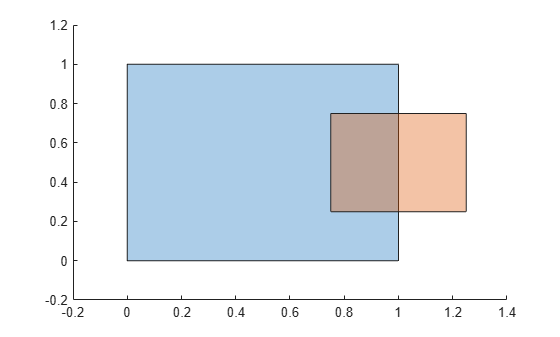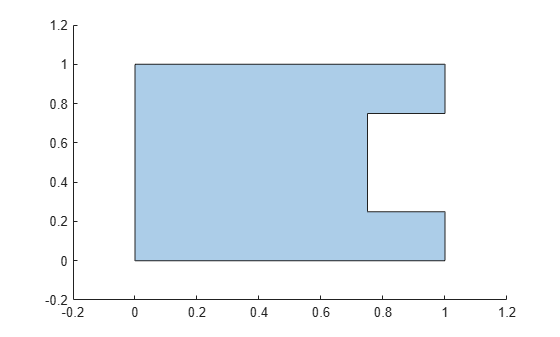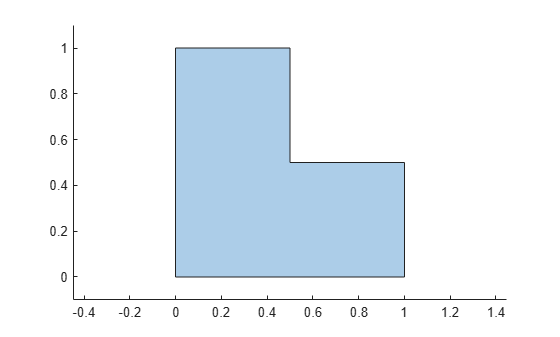# subtract

Difference of two `polyshape` objects

## Syntax

``polyout = subtract(poly1,poly2)``
``[polyout,shapeID,vertexID] = subtract(poly1,poly2)``
``___ = subtract(poly1,poly2,'KeepCollinearPoints',TF)``

## Description

example

````polyout = subtract(poly1,poly2)` returns a `polyshape` object whose regions are the geometric difference of two `polyshape` objects. The output `polyout` contains the regions of `poly1` minus any part of `poly2` that overlaps with `poly1`. The input arguments `poly1` and `poly2` must have compatible array sizes.```

example

````[polyout,shapeID,vertexID] = subtract(poly1,poly2)` also returns vertex mapping information from the vertices in `polyout` to the vertices in `poly1` and `poly2`. The `subtract` function only supports this syntax when `poly1` and `poly2` are scalar `polyshape` objects.The `shapeID` elements identify whether the corresponding vertex in `polyout` originated in `poly1`, `poly2`, or was created from the difference. `vertexID` maps the vertices of `polyout` to the vertices of `poly1`, `poly2`, or the difference.```
````___ = subtract(poly1,poly2,'KeepCollinearPoints',TF)` specifies whether to keep or remove collinear points in `polyout` for any of the previous syntaxes.```

## Examples

collapse all

Create and plot two polygons `poly1` and `poly2` that partially overlap.

```poly1 = polyshape([0 0 1 1],[1 0 0 1]); poly2 = polyshape([0.75 1.25 1.25 0.75],[0.25 0.25 0.75 0.75]); plot(poly1) hold on plot(poly2)````figure`

Subtract `poly2` from `poly1`. The resulting polygon is `poly1` minus any part of `poly2` that overlaps with `poly1`.

`polyout1 = subtract(poly1,poly2)`
```polyout1 = polyshape with properties: Vertices: [8x2 double] NumRegions: 1 NumHoles: 0 ```
`plot(polyout1)`Now subtract the two polygons in the opposite order, that is, subtract `poly1` from `poly2`. The resulting polygon is `poly2` minus any part of `poly1` that overlaps `poly2`.

`polyout2 = subtract(poly2,poly1)`
```polyout2 = polyshape with properties: Vertices: [4x2 double] NumRegions: 1 NumHoles: 0 ```
```plot(polyout2) xlim([-0.2 1.4]); ylim([-0.2 1.2]);```Create two polygons, and compute and plot their difference. Display the vertex coordinates of the difference and the corresponding vertex mapping information.

```poly1 = polyshape([0 0 1 1],[1 0 0 1]); poly2 = translate(poly1,[0.5 0.5]); [polyout,shapeID,vertexID] = subtract(poly1,poly2); plot(polyout) axis equal````[polyout.Vertices shapeID vertexID]`
```ans = 6×4 0 1.0000 1.0000 1.0000 0.5000 1.0000 0 0 0.5000 0.5000 2.0000 4.0000 1.0000 0.5000 0 0 1.0000 0 1.0000 3.0000 0 0 1.0000 4.0000 ```

The first, fifth, and sixth vertices of the difference originated in `poly1`, since the corresponding values in `shapeID` are 1. These vertices are the first, third, and fourth vertices in the property `poly1.Vertices`, respectively, since the corresponding values in `vertexID` are 1, 3, and 4. Similarly, the third vertex of the difference originated in `poly2`, and is the fourth vertex in the property `poly2.Vertices`. The second and fourth vertices of the difference were created from the subtraction computation because the corresponding values of `shapeID` and `vertexID` are 0.

## Input Arguments

collapse all

First input `polyshape`, specified as a scalar, vector, matrix, or multidimensional array.

Second input `polyshape`, specified as a scalar, vector, matrix, or multidimensional array.

Collinear vertices indicator, specified as `false` or `true`:

• `false` — Remove collinear points so that the output `polyshape` contains the fewest vertices necessary to define the boundaries.

• `true` — Keep all collinear points as vertices.

When the `'KeepCollinearPoints'` parameter is not specified, its value is assigned according to the values used when creating the input `polyshape` objects:

• If the value was `true` for each input `polyshape` when they were created, then the value for the output `polyshape` is set to `true`.

• If the value was `false` for each input `polyshape` when they were created, then the value for the output `polyshape` is set to `false`.

• If the values for the input `polyshape` objects do not match, then the value for the output `polyshape` is set to `false`.

Data Types: `logical`

## Output Arguments

collapse all

Output `polyshape`, returned as a scalar, vector, matrix, or multidimensional array.

The two input `polyshape` arguments must have compatible sizes. For example, if two input `polyshape` vectors have different lengths M and N, then they must have different orientations (one must be a row vector and one must be a column vector). `polyout` is then M-by-N or N-by-M depending on the orientation of each input vector. For more information on compatible array sizes, see Compatible Array Sizes for Basic Operations.

Shape ID, returned as a column vector whose elements each represent the origin of the vertex in the difference. The value of an element in `shapeID` is 0 when the corresponding vertex of the output `polyshape` was created by the subtraction. An element is 1 when the corresponding vertex originated from `poly1`, and 2 when it originated from `poly2`.

The length of `shapeID` is equal to the number of rows in the `Vertices` property of the output `polyshape`. The `xor` function only supports this output argument if the input `polyshape` objects are scalar.

Data Types: `double`

Vertex ID, returned as a column vector whose elements map the vertices in the output `polyshape` to the vertices in the `polyshape` of origin. The elements of `vertexID` contain the row numbers of the corresponding vertices in the `Vertices` property of the input `polyshape`. An element is 0 when the corresponding vertex of the output `polyshape` was created by the difference.

The length of `vertexID` is equal to the number of rows in the `Vertices` property of the output `polyshape`. The `subtract` function only supports this output argument when the input `polyshape` objects are scalar.

Data Types: `double`